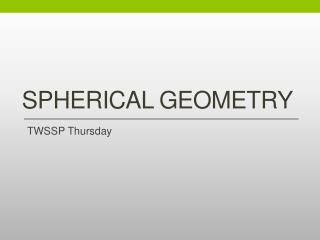DownloadDownload PresentationSpherical Geometry

# Spherical Geometry

Télécharger la présentation## Spherical Geometry

- - - - - - - - - - - - - - - - - - - - - - - - - - - E N D - - - - - - - - - - - - - - - - - - - - - - - - - - -
##### Presentation Transcript

1. Spherical Geometry TWSSP Thursday

3. Thursday Agenda • Agenda • Further investigate spherical lines • Investigate distances on the sphere • Investigate spherical polygons • Questions for today: What are the spherical analogues of common Euclidean figures? How do taxicab and spherical geometries compare to Euclidean? • Success criteria: I can … compare and contrast taxicab and Euclidean geometries and spherical and Euclidean geometries.

4. A question from yesterday • Is a line infinite in spherical geometry? Think – Go Around - Discuss

5. Distances • The Earth as a sphere in Euclidean space has a radius of 6,400 km i.e. the radius as measured from the center of the sphere to any point on the surface of Earth is 6,400 km • What is Earth’s circumference? • How many degrees does this represent? • If two places on Earth are opposite each other, what is the distance between them in kilometers in the spherical sense? In degrees? • If two places are 90o apart from each other, how far apart are they in kilometers in the spherical sense? • If two places are 5026 km apart, how far apart are they in degrees?

6. Distances • Mars has a circumference of 21,321 kilometers. What does this distance represent in degrees? • What is the furthest distance that two places on Mars can be apart from each other in degrees? In kilometers (in the spherical sense)? • What is the minimum information we need to find the distance between two points on a sphere? • Is there a distance formula?

7. Special Lines • Yesterday, you conjectured that there are no parallel lines on the sphere. Can you justify this conjecture? • What are perpendicular lines on the sphere? • Given a line and a point in the Euclidean plane, how many perpendiculars can you draw through that point? • Investigate the same idea on the sphere. Does the location of the point matter?

8. More Perpendicular Lines • Given any two lines in the plane, how many lines can you construct which are perpendicular to both of them? • Given any two lines on the sphere, how many lines can you construct which are perpendicular to both of them?

9. Figures on the Sphere • What is the minimum number of sides required to make a closed figure in the Euclidean plane? • What is the minimum number of sides required to make a closed figure on the sphere?

10. Spherical Triangles • Given any three non-collinear points in the plane, how many triangles can you form between those points? • Given any three non-collinear points on the sphere, how many triangles can you form between those points? • What is the sum of the angles of a Euclidean-triangle? How do you know? • What is the sum of the angles of a spherical-triangle? How do you know?

11. Squares • Investigate squares on the sphere. Justify any conclusions you make. • Record your conclusions on your whiteboard and prepare to share out.

12. Comparing Geometries • Choose a partner • You will be assigned 2 or 3 ideas to compare to corresponding ideas in Euclidean geometry. For example, if you are asked to compare taxicab and Euclidean circles, you should identify all the ways in which they are similar, and all the ways in which they are different. • You will each report out to a new partner, receive feedback on your comparisons, and return to your original partner • You will make a poster to present to the class which shares your comparisons.

13. Wrap up • What questions do you have left about either of the geometries? • What questions do you have about teaching geometry?